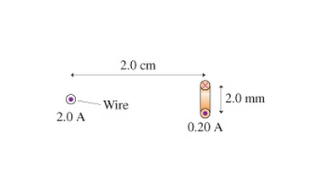# Problem: (a) What is the magnitude of the torque on the current loop in the figure? Express your answer using two significant figures.(b) What is the loop's equilibrium orientation?A. verticalB. horizontal

###### FREE Expert Solution

(a) Torque is expressed as:

$\overline{){\mathbf{\tau }}{\mathbf{=}}{\mathbf{NBAI}}{\mathbf{s}}{\mathbf{i}}{\mathbf{n}}{\mathbf{\theta }}}$

82% (9 ratings)###### Problem Details(a) What is the magnitude of the torque on the current loop in the figure? Express your answer using two significant figures.

(b) What is the loop's equilibrium orientation?

A. vertical

B. horizontal